# 2014 A-level H2 Mathematics (9740) Paper 1 Question 6 Suggested SolutionsAll solutions here are SUGGESTED. Mr. Teng will hold no liability for any errors. Comments are entirely personal opinions.

(a)
(i)
Let P(n) be the preposition,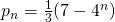forWhenandSince, P(1) is true.
Assume P(k) is true for some.
To prove that P(K+1) is true, i.e.,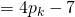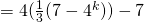Therefore, P(k) is trueP(k+1) is also true.
Since P(1) is true and P(k) is trueP(k+1) is true, by principle of mathematical induction, P(n) is true for all.

(ii)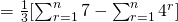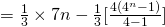(b)
(i)
AsTherefore, the series converges and the.

(ii)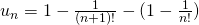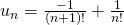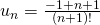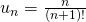Convergence of a series can be proved in a few ways, the given is the most direct, which is to show that the sum to infinity is a finite value. Question wants students to write down, so please write it explicitly. It is surprising that some students confuse the formulas and write, this is totally wrong.
Not readable? Change text.